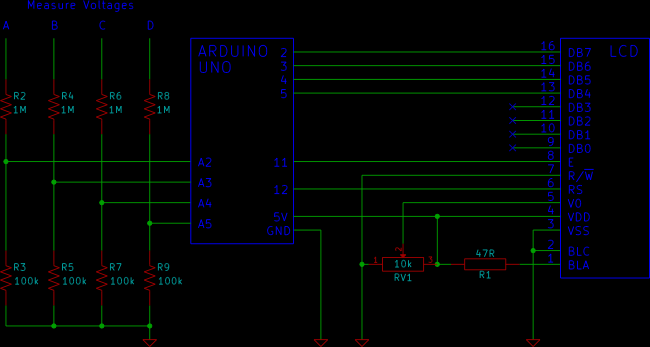# Arduino LCD Voltmeter with 4 Channels

Analog channels A2 to A5 on an Arduino Uno are used to measure four different voltages. The measured voltages are displayed on a 16 character by 2 line LCD.

The four channel Arduino multimeter can measure four independent DC voltages that can each be in the range of 0 to 50V.

Voltages are displayed with one decimal place, e.g. 5.3V, 12.8V, etc.This video shows the Arduino voltmeter being used to measure the voltage of four batteries. Each battery has a different voltage.

Can’t see the video? View on YouTube →

## How the Voltmeter Works

Each channel of the Arduino voltmeter has a pair of resistors that form a voltage divider. The voltage divider scales down the input voltage to a range that the Arduino can measure. Code running on the Arduino calculates the actual voltage and displays the result on the LCD.

How the voltage divider works, precautions and voltage range are explained in the article Measuring DC Voltage using Arduino. The Arduino voltmeter uses the same resistor values as the article.

## Arduino Voltmeter Circuit Diagram

The LCD used in the circuit has the pin numbering shown in the LCD component information from the beginner’s tutorial on this website.

It is important to check that the LCD that you are using has the same pin numbering as the circuit diagram. Making incorrect LCD connections can damage the LCD.

If you are using a LCD with different pin numbering, use the pinout information from your LCD’s datasheet to make the connections to the Arduino.

The Arduino LCD tutorial explains how to connect a LCD to an Arduino Uno. The soldering article has a video that shows how to connect a pin header to the LCD so that it can be plugged into a breadboard.

Voltages are measured between points A, B, C or D and GND or 0V. Remember to adjust the contrast pot. so that the voltages on the LCD are made visible.

Resistor R1 provides current limiting for an optional backlight and keeps the backlight permanently on.

## Arduino Voltmeter Sketch Code

The sketch is based on the code from the Measuring DC Voltage using Arduino article.

The sum and voltage variables have been changed into arrays so that they can store values from four analog channels. The voltage calculations work the same way as the original sketch, but now do the calculations for four channels.

For more detail: Arduino LCD Voltmeter with 4 Channels

#### This Post / Project can also be found using search terms:

• 4 chAnnel i2c lcd voltmeter
• arduino 4 channel multimeter
• Arduino lcd voltmeters
• down loadable arduino 4 chanal volt meater codes